Next: 2.5 Measuring the Characteristic Up: 2 The Characteristic Length Previous: 2.3.3 Nonlinear and Nonlocal

# 2.4 The GL Penetration Depth and Coherence Length

The temperature and magnetic field dependence of both the penetration depth and coherence length appear quite naturally in Ginzburg-Landau (GL) theory . Like the London model, the GL model is independent of the underlying mechanism for superconductivity. However, it must be emphasized that GL theory is strictly valid only near the normal-to-superconducting phase boundary, and is thus not generally applicable at low temperatures. In the theory, a complex order parameter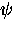is introduced, whereis a function of temperature, magnetic field and the spatial coordinates. Ginzburg and Landau assumed that near Tc whereis small, the free-energy difference per unit volume between the normal and superconducting state at zero magnetic field may be expanded as a function of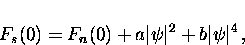(50)
where a and b are temperature-dependent coefficients such that near Tc

where a0 and b0 are positive coefficients, so that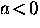below Tc. Minimizing the free energy with respect to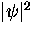gives the zero-field value of the order parameter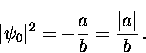(51)

In the presence of a magnetic field, the free energy in the superconducting state is increased. In particular, the work done on the sample by the magnetic field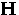is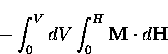(52)
where V is the volume of the sample and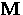is the sample magnetization. In the Meissner phase,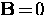so that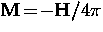, whereas in the normal stateis essentially zero. This means that the Gibbs free energy per unit volume in the superconducting state increases in the presence of a magnetic field to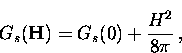(53)
whereas in the normal state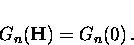(54)
At the phase change,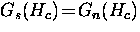where Hc is the critical field. Thus, using Eq. (2.56) and Eq. (2.57), one has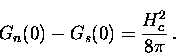(55)
Using the Legendre transformation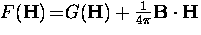,Eq. (2.58) may be written in terms of the Helmholtz free energy per unit volume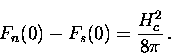(56)
This implies that an energy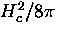, called the condensation energy'', is given up by the formation of the superconducting state.

Assuming that the order parameteris not completely rigid in the presence of a magnetic field, there is an additional energy term associated with variations in.This term was assumed by Ginzburg and Landau to take the form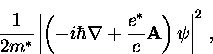(57)
where later it was found that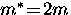and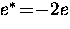.Thus, the total free energy per unit volume of the superconducting state in the presence of a magnetic field is

Minimizing this expression with respect toleads to the first GL equation''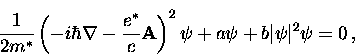(58)
and with respect to, the second GL equation''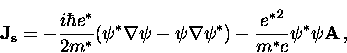(59)
which is the standard expression for the quantum-mechanical current. This equation has the same form as the London equation, except thatis spatially varying. The GL equations can be solved analytically for simple cases only.

Consider a superconductor in a weak magnetic field with the sample dimensions much greater than the magnetic penetration depth. To first order in B,in Eq. (2.63) can be replaced by its equilibrium zero-field value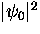from Eq. (2.54)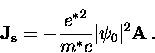(60)
Taking the curl of both sides of this equation gives(61)
Using Eq. (2.12), this can be rewritten as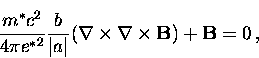(62)
which, upon comparing to the expression in Eq. (2.13), gives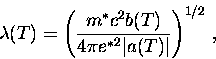(63)
which is the same as the London penetration depth if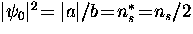.

Consider a second example wherevaries only in the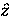-direction, but the applied magnetic field is zero. In this case the first GL equation becomes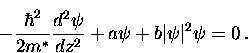(64)
Assumingis real, we can introduce a dimensionless order parameter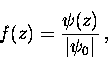(65)
where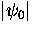is given by Eq. (2.54). Thus, Eq. (2.68) becomes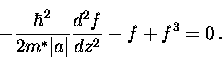(66)
A natural length scale for spatial varitaions of the order parameter is thus(67)
which is known as the GL coherence length. Note that both the GL coherence length and the GL penetration depth are temperature dependent quantities. From Eq. (2.53) it is clear that both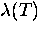and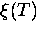vary as (1-T/Tc)-1/2 with temperature, so that their ratio is independent of temperature(68)is known as the GL parameter''. A precise calculation from the microscopic theory gives a weak temperature dependence for, withincreasing as T decreases . In GL theory, a superconductor is called type-I'' if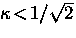and type-II'' if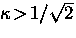.When the order parameter throughout the sample is essentially a constant, the GL model reduces to the London model. This occurs when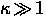, which is the case for the high-Tc superconductors.

GL theory is particularly useful in modelling the small variation of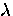with magnetic field found near Tc. Pippard  first used a simple thermodynamic argument, which distributes the entropy difference due to changes in H over a layer of thickness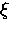, to explain the field dependence ofhe observed in the type-I superconductor Sn. Historically, this marks the introduction ofas a fundamental length scale in superconductivity theory. Although the field dependence is built into the GL theory, for arbitrary H,has a non-negligible dependence on field. Thus, in general, the field dependence of bothandmust be obtained by numerically solving the complete nonlinear GL equations. Analytical results can be obtained in special cases, such as for thin films.Next: 2.5 Measuring the Characteristic Up: 2 The Characteristic Length Previous: 2.3.3 Nonlinear and Nonlocal